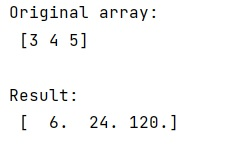# Factorial in numpy and scipy

Learn, how to calculate factorial of a number in numpy and scipy?
Submitted by Pranit Sharma, on January 20, 2023

NumPy is an abbreviated form of Numerical Python. It is used for different types of scientific operations in python. Numpy is a vast library in python which is used for almost every kind of scientific or mathematical operation. It is itself an array which is a collection of various methods and functions for processing the arrays.

## Finding the factorial in numpy and scipy

SciPy is a free and open-source Python library used for scientific computing and technical computing. We can use SciPy modules for optimization, interpolation, special functions, linear algebra, integration FFT, signal and image processing, ODE solvers, and other tasks common in science and engineering.

Suppose that we are given a NumPy array and we need to import numpy and scipy factorial functions to check which one works faster.

Basically, scipy.math.factorial(), numpy.math.factorial(), and math.factorial() are same when it comes to their processing. However, scipy.special.factorial is different as it can take a ndarray as an input while others can't.

Let us understand with the help of an example,

## Python code to find the factorial in numpy and scipy

```# Import numpy
import numpy as np

# Import scipy special
import scipy.special

# Creating a numpy array
arr = np.array([3, 4, 5])

# Display original array
print("Original array:\n",arr,"\n")

res = scipy.special.factorial(arr)

# Display the result
print("Result:\n",res)
```

Output:What's New (MCQs)

Top Interview Coding Problems/Challenges!

IncludeHelp's Blogs

Languages: » C » C++ » C++ STL » Java » Data Structure » C#.Net » Android » Kotlin » SQL
Web Technologies: » PHP » Python » JavaScript » CSS » Ajax » Node.js » Web programming/HTML
Solved programs: » C » C++ » DS » Java » C#
Aptitude que. & ans.: » C » C++ » Java » DBMS
Interview que. & ans.: » C » Embedded C » Java » SEO » HR
CS Subjects: » CS Basics » O.S. » Networks » DBMS » Embedded Systems » Cloud Computing
» Machine learning » CS Organizations » Linux » DOS
More: » Articles » Puzzles » News/Updates